# MP Board Class 11th Maths Important Questions Chapter 15 Statistics

## MP Board Class 11th Maths Important Questions Chapter 15 Statistics

### Statistics Important Questions

Question 1.
Find the mean deviation about the mean for the data in following table: (NCERT)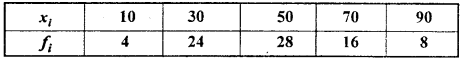Solution:Mean $$\bar { x }$$ = $$\frac{\sum f_{i} x_{i}}{\sum f_{i}}$$ = $$\frac { 4000 }{ 80 }$$ = 50
Mean deviation about mean = $$\frac{\sum f_{i}\left|x_{i}-\bar{x}\right|}{\sum f_{i}}$$
= $$\frac { 1280 }{ 80 }$$ = 16.

You can use this Standard Deviation Calculator to calculate the standard deviation, variance, mean, and the coefficient of variance for a given set .

Question 2.
Find the mean deviation about the median for the data in following table: (NCERT)Solution:$$\frac { N }{ 2 }$$ = $$\frac { 26 }{ 2 }$$ = 13
Which lies on cumulative frequency (C.F.) 14.
∴ Median = Md = 7.
Mean deviation about mean = $$\frac{\sum f_{i}\left|x_{i}-M_{d}\right|}{\sum f_{i}}$$
= $$\frac { 1128.8 }{ 100 }$$

Question 3.
Find the mean deviation about the mean for the data in following table: (NCERT)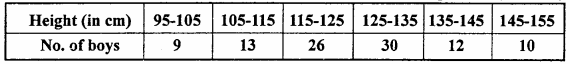Solution:
Let A = 130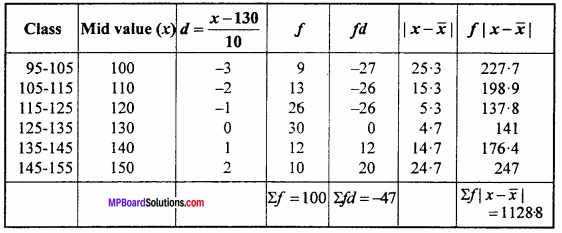Mean $$\bar { x }$$ = A + $$\frac { Σfd }{ Σf }$$ × i = 130 + $$\frac { (- 47) }{ 10 }$$ = 10
$$\bar { x }$$ = 130 – 4.7 = 125.3
Mean deviation about mean = $$\frac{\sum f_{i}\left|x_{i}-\bar{x}\right|}{\sum f_{i}}$$
= $$\frac { 1128.8 }{ 100 }$$ = 11.228.

Question 4.
Find the mean deviation about the median for the data in following table: (NCERT)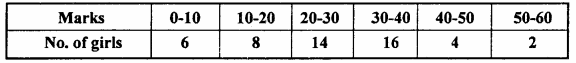Solution:$$\frac { N }{ 2 }$$ = $$\frac { 50 }{ 2 }$$ = 25
20 – 30 is median class.
Here F = 14, f = 14, l = 20, i = 10Question 5.
Calculate the mean deviation about median for the age distribution of 100 persons given below :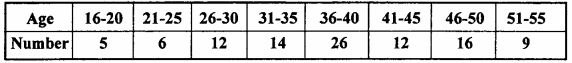Solution:
First make the class interval uniform: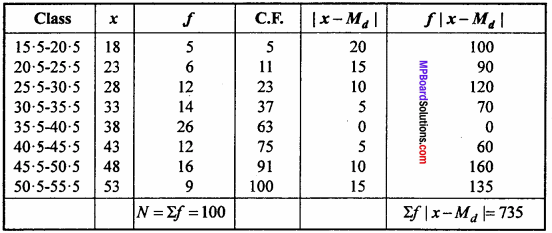$$\frac { N }{ 2 }$$ = $$\frac { 100 }{ 2 }$$ = 50
Median class = 35.5 – 40.5
where l = 35.5, F = 37, f = 26, i = 5Question 6.
Find the mean and variance for the following frequency distribution in following table:
(A)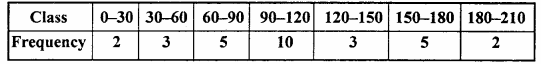Solution:Let assumed mean A = 105(B)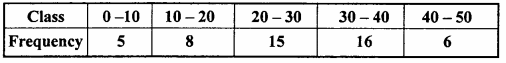Solution:Let assumed mean A = 25Question 7.
Find the mean and variance and standard deviation using short cut method:Solution: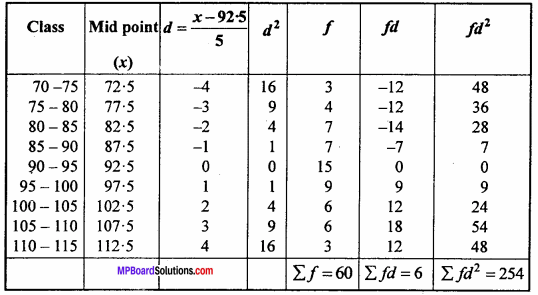Let assumed mean A = 92.5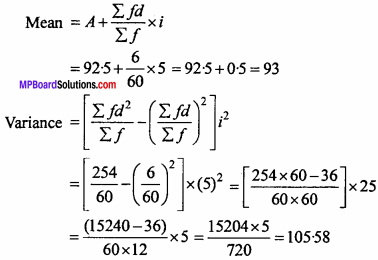Standard deviation σ = $$\sqrt {variance}$$
= $$\sqrt {105.58}$$ = 10.27

Question 8.
The diameter of circle (in mm) drawn in design are given below :Calculate the standard deviation and mean diameter of the circles. (NCERT)
Solution:
First make the class interval uniform :Let assume mean A = 42.5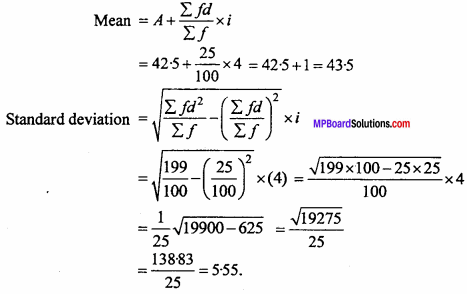Question 9.
From the prices of shares X and Y below, find out which is more stable in value: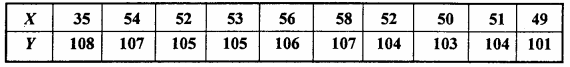Solution:
For first share X : Let assumed mean A = 58, n = 10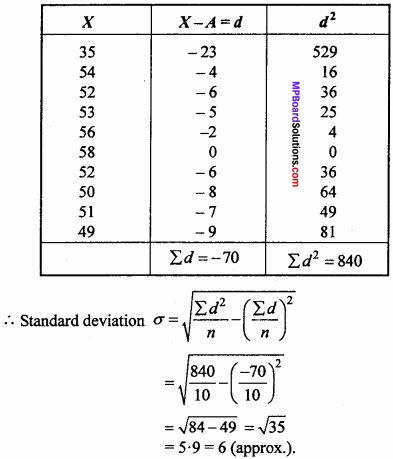Foe second share Y : Let assumed mean A = 107, n = 10Coefficient of variation for X :
Mean M = A + $$\frac { Σd }{ n }$$
= 58 + $$\frac { ( – 70) }{ 10 }$$ = 58 – 7
= 51
∴ Coefficient of variance = $$\frac { σ }{ M }$$ x 100
= $$\frac { 6 }{ 51 }$$ x 100 = 11.8
Coefficient of variance of Y :
Mean M = A + $$\frac { Σd }{ n }$$
= 107 + $$\frac { – 20 }{ 10 }$$ = 107 – 2
= 105
∴ Coefficient of variation = $$\frac { σ }{ M }$$ x 100
= $$\frac { 2 }{ 105 }$$ x 100 = 1.9
Coefficient of variance of Y is less than the coefficient of variance of X.
Share Y is more stable than share X.

Question 10.
An analysis of monthly wages paid to the workers in two firms A and B belonging to the same industry. Give the following results: (NCERT)1. Which firm A or B pays out larger amount as monthly wages.
2. Which firm A or B shows greater variability in individual wages.

Solution:
For firm A :
Number of wages earners = 586
Mean of monthly wages x = Rs. 5,253
Amount paid by firm A = 586 x 5,253 = Rs. 30, 78, 258
Variance of distribution of wages = 100
Standard deviation σ = $$\sqrt {100}$$ = 10
Coefficient of variation = $$\frac{\sigma}{\bar{x}}$$ x 100 = $$\frac { 10 }{ 5253 }$$ = 0.19

For firm B :
Number of wages earners = 648
Mean $$\bar { x }$$ = Rs. 5253
Amount paid by firm B = 648 x 5253 = Rs. 34, 03, 944
Coefficient of variation = 121
Standard deviation σ = $$\sqrt {121}$$ = 11
Coefficient of variation = $$\frac{\sigma}{\bar{x}}$$ x 100 = $$\frac { 11 }{ 5253 }$$ x 100 = 0.21
Thus, firm B pays out larger amount as monthly wages.
∵ C.V. of firm B > C.V. of firm A.
∴ Firm B shows greater variability in individual wages.

Question 11.
The following is the record of goals scored by team A in a football session:For the team B, mean number of goals scored per match was 2 with a standard deviation 1.25 goals. Find which team may be considered more consistent? (NCERT)
Solution:For team B :C.V of team A < C.V of team B. Hence team A is more consistent.

Question 12.
The mean and standard deviation of marks obtained by 50 students of three subjects Mathematics, Physics and Chemistry are given below :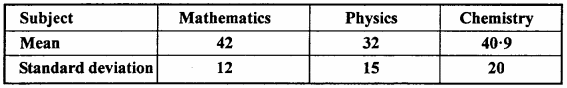Which of these three subjects shows the highest variability in marks and which shows lowest? (NCERT)
Solution:
C.V. of Mathematics (C.V.) = $$\frac{\sigma}{\bar{x}}$$ x 100
Where σ = 12, $$\bar { x }$$ = 42
∴ C.V. of Mathematics = $$\frac { 12 }{ 42 }$$ x 100 = 28.57
C.V. of Physics = $$\frac{\sigma}{\bar{x}}$$ x 100
Where σ = 15, $$\bar { x }$$ = 32
∴ C.V. of Physics = $$\frac { 15 }{ 32 }$$ x 100 = 46.87
C.V. of Chemistry = $$\frac{\sigma}{\bar{x}}$$ x 100
Where σ = 20, $$\bar { x }$$ = 40.9
∴ C.V. of Chemistry = $$\frac { 20 }{ 40.9 }$$ x 100
= 48.89
∵ C.V. of Chemistry > C.V. of Physics > C.V. of Mathematics.
∴ Chemistry shows the highest variability and Mathematics shows the least variability.

Question 13.
The mean and standard deviation of a group of 100 observations were found to be 20 and 3 respectively. Later on it was found that three observations were incorrect Which are recorded by 21,21, and 18. Find the mean and standard deviation, if the incorrect observations are omitted. (NCERT)
Solution:
Given: n = 100, $$\bar { x }$$ = 20, σ = 3
$$\bar { x }$$ = $$\frac{\sum x}{n}$$ ⇒ 20 = $$\frac{\sum x}{n}$$
Σx = 20 x 100 = 2000
If incorrect observations 21,21 and 18 are omitted, then correct sum
Σx = 2000 – 21 – 21 – 18 = 2000 – 60 = 1940
Now correct mean of remaining 97 observations are
$$\bar { x }$$ = $$\frac{\sum x}{n}$$ = $$\frac { 1940 }{ 97 }$$ = 20
Given : σ = 3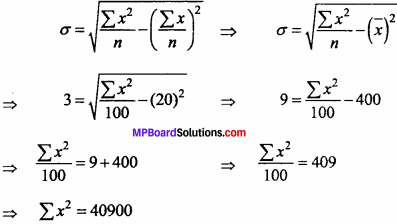correct Σx2 = 40900 – (21)2 – (21)2 – (18)2
= 40900 – 441 – 441 – 324 = 39694
Now correct S.D. of remaining 97 observations are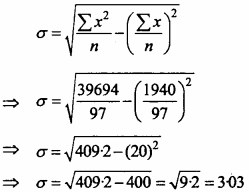Question 14.
The mean and variance of eight observations are 9 and 9.25 respectively. If six of the observations are 6, 7, 10, 12, 12 and 13, then And the remaining two observations. (NCERT)
Solution:
Let the two remaining observations are x1 and x2
Mean M = $$\frac{6 + 7 + 10 + 12 + 12 + 13 + x_{1} + x_{2}}{8}$$
9 = $$\frac{60 + x_{1} + x_{2}}{8}$$
⇒ x1 + x2 + 60 = 72
⇒ x1 + x2 = 12 …. (1)⇒ x21 + x22 = 722 – 642
⇒ x21 + x22 = 80 …. (2)
From eqn. (1),
x2 = 12 – x1
Put the value of x2 in equation (2),
x21 + (12 – x1)2 = 80
⇒ x2 + 144 + x21 – 24x1 = 80
⇒ 2x21 – 24x1 + 64 = 0
⇒ x21 – 12x1 + 32 = 0
⇒ x21 – 4x1 – 8x1 + 32 = 0
⇒ x1(x1 – 4) – 8(x1 – 4) = 0
⇒ (x1 – 8)(x1 – 4) = 0
⇒ x1 = 4, 8
When x1 = 4, then x2 = 12 – 4 = 8
When x1 = 8, then x2 = 12 – 8 = 4
Hence remaining observations are 4 and 8.